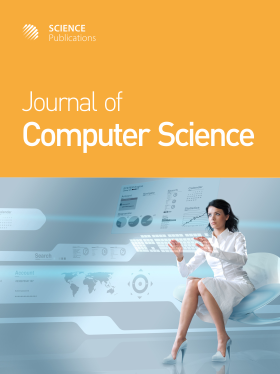Frequency: Monthly
ISSN: 1549-3636 (Print)
ISSN: 1552-6607 (Online)
Research Article Open Access

# A Reduced Finite Element Model for Analyzing the Transverse Shear Stiffness of Truss-Like Core Sandwich Beam

Suphattharachai Chomphan and Manit Leekitwattana

## Abstract

Problem statement: In structural analysis using finite element software, a number of finite element equations affect the speed of calculation time. A reduced finite element model should be used for analyzing structural responses to reduce the calculation time. Approach: This study presents the analysis of transverse shear stiffness of truss-like core sandwich beam using the reduced finite element model based on a unit cell approach. Two kinds of core topologies; an X-truss core and a bi-directional X-truss core, were chosen to be analyzed. The presented reduced finite element model based on the unit cell approach was compared in transverse shear stiffness with the conventional three-point loaded beam approach. Results: Results showed that the unit cell approach could be used for analyzing the transverse shear stiffness of truss-like core sandwich beam with a good correlative with the conventional three-point loaded beam approach and a significantly reduced number of finite element equations. Conclusion: The transverse shear stiffness of truss-like core sandwich beam can be obtained from the reduced finite element model based on unit cell approach. This reduced finite element model can be used to reduce the number of finite element equations; consequently, the speed of calculation time is increased.

Journal of Computer Science
Volume 7 No. 10, 2011, 1474-1477

DOI:

Submitted On: 12 April 2011 Published On: 4 August 2011

How to Cite: Chomphan, S. & Leekitwattana, M. (2011). A Reduced Finite Element Model for Analyzing the Transverse Shear Stiffness of Truss-Like Core Sandwich Beam. Journal of Computer Science, 7(10), 1474-1477. https://doi.org/10.3844/jcssp.2011.1474.1477

• 1,780 Views
• 0 Citations